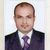# App Development

Discussion board where members can learn more about Qlik Sense App Development and Usage.

Announcements
April 22, 2PM EST: Learn about GeoOperations in Qlik Sense SaaS READ MORE
cancel
Showing results for
Search instead for
Did you mean:Contributor II

## Data Load Editor Invalid Expression

Hi,

I am trying to use this expression in the data load editor to create a new table but I keep getting the invalid expression error.

If((1-(Sum(If(month= currentmonth, Spend))/Sum(If(month= currentmonth, Forecast)))) > .20, 'Red',

If((1-(Sum(If(month= currentmonth, Spend))/Sum(If(month= currentmonth, Forecast)))) > - .20, 'Green')) as RAG

What would need to be changed to make this able to work.

Regards,

William

1 Solution

Accepted SolutionsContributor II
Author

I solved the issue by removing the sum from the expression.

Thanks.

4 RepliesCreator II

Hi William,

Please share the complete load script for this expression. If you are using any aggregation function in script like Sum you need to do group by for all the fields that you are loading from the table.

BR,

NehaMVP

Remove the space between the - and the .20

If(1 - Sum(If(month = currentmonth, Spend)) / Sum(If(month= currentmonth, Forecast)) > .20, 'Red',

If(1 - Sum(If(month = currentmonth, Spend)) / Sum(If(month = currentmonth, Forecast)) > -.20, 'Green')) as RAG

^^^

Logic will get you from a to b. Imagination will take you everywhere. - A EinsteinMVP

What error message you getting?

Try to write this way

If((1 - (Sum(If(month= currentmonth, Spend)) / Sum(If(month= currentmonth, Forecast)))) > 0.20, 'Red',

If((1 - (Sum(If(month= currentmonth, Spend)) / Sum(If(month= currentmonth, Forecast)))) > - 0.20, 'Green')) as RAGContributor II
Author

I solved the issue by removing the sum from the expression.

Thanks.